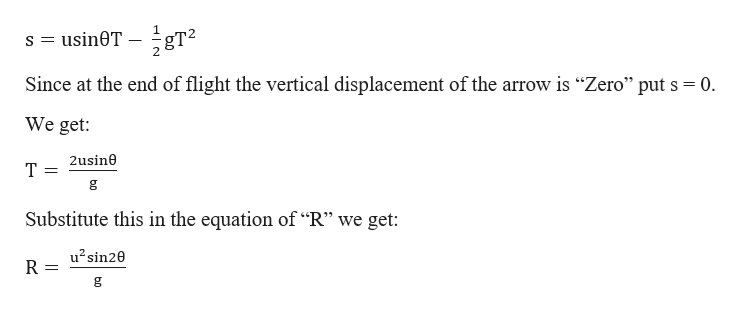# William Tell is said to have shot an apple off his son’s head with an arrow. If the arrow was shot with an initial speed of 50.0 m/s and the boy was 26.0 m away, at what launch angle above the horizontal did Bill aim the arrow? (Assume that the arrow and apple are initially at the same height above the ground. Hint: sin2θ = 2sinθcosθ)

Question

William Tell is said to have shot an apple off his son’s head with an arrow. If the arrow was shot with an initial speed of 50.0 m/s and the boy was 26.0 m away, at what launch angle above the horizontal did Bill aim the arrow? (Assume that the arrow and apple are initially at the same height above the ground. Hint: sin2θ = 2sinθcosθ)

check_circleExpert Solution
Step 1

Given information:

The apple and the arrow are at the same height before launch.

The initial speed of the arrow (u) = 50 m/s

Distance between the apple and the arrow (R) = 26 m.

Angle of launch (θ) =?

Step 2

We can say that the distance travelled by the arrow launched with an angle (θ) is given by:

R = ucosθ × (time of flight(T))

But “T” for a projectile is given from second equation of motion as follows:help_outlineImage TranscriptioncloseusineT Since at the end of flight the vertical displacement of the arrow is "Zero" put s 0 We get 2usine T g Substitute this in the equation of "R" we get: u2 sin20 R g fullscreen
Step 3

Calculation:

For the arrow to hit the apple the condition is R = 26 m, hen...

### Want to see the full answer?

See Solution

#### Want to see this answer and more?

Solutions are written by subject experts who are available 24/7. Questions are typically answered within 1 hour*

See Solution
*Response times may vary by subject and question
Tagged in

### Kinematics